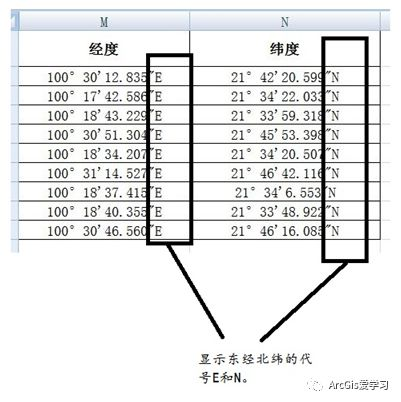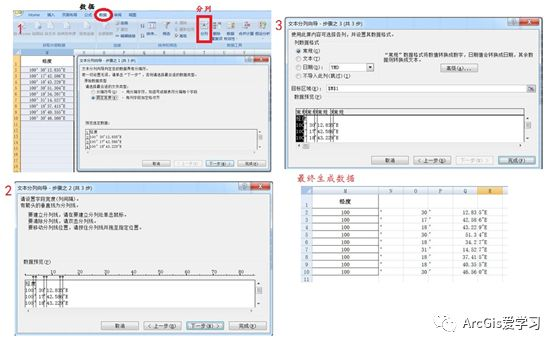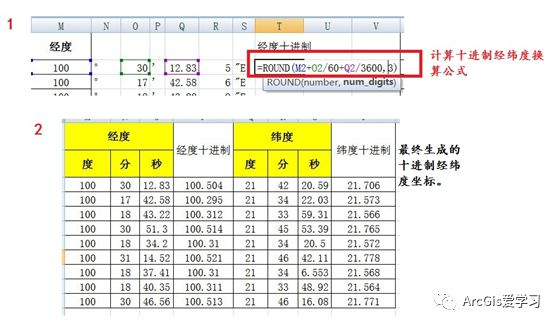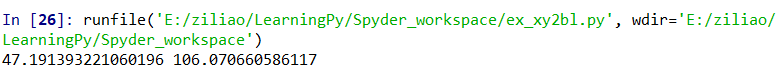• wgs84平面坐标转经纬度
千次阅读
2019-11-25 23:15:08

# ECEF坐标系转经纬度 wgs84 - 方法提炼

本文提供坐标系转换算法，根据某前端算法修改完善为C#语言支持。

最近在项目中遇到空间坐标转换问题，现提供相关算法C#支持。

### 源代码

相关代码如下：

using System;

namespace ConsoleApp1
{
/// <summary>
/// ECEF坐标系转换经纬度 wgs84
/// 方法提炼
/// </summary>
internal class Program
{
private static void Main(string[] args)
{
double[] ret = XYZ2LLA(new double[]
{
3333,4444,1500
});

Console.WriteLine(string.Format("LON-:{0}\r\nLAT-:{1}\r\nALT-:{2}\r\n", ret, ret, ret));

}

private static double EARTH_1 = 6378.137;
private static double Temp_1 = EARTH_1 * (1.0 - (1.0 / 298.257223563));
private static double Eccsq = 1 - Math.Pow(Temp_1, 2) / Math.Pow(EARTH_1, 2);
private static double Dtr = Math.PI / 180.0;

/// <summary>
/// ECEF坐标系转经纬度 wgs84
/// 输入-千米、千米、千米
/// 输出-经、纬、千米
/// </summary>
/// <param name="xvec"></param>
/// <returns></returns>
private static double[] XYZ2LLA(double[] xvec)
{
double flatgc, flatn, dlat, rp, x, y, z, p, tangd, rn, clat, slat, flat, flon, altkm;
x = xvec;
y = xvec;
z = xvec;

rp = Math.Sqrt(Math.Pow(x, 2) + Math.Pow(y, 2) + Math.Pow(z, 2));
flatgc = Math.Asin(z / rp) / Dtr;
flon = ((Math.Abs(x) + Math.Abs(y)) < 1.0e-10) ? 0.0 : Math.Atan2(y, x) / Dtr;
flon = (flon < 0.0) ? flon + 360.0 : flon;
p = Math.Sqrt(Math.Pow(x, 2) + Math.Pow(y, 2));

if (p < 1.0e-10)
{
flat = ((z < 0.0)) ? -90.0 : 90.0;
}
else
{
flat = Gc2gd(flatgc, altkm);
for (double kount = 0; kount < 5; kount++)
{
slat = Math.Sin(Dtr * flat);
tangd = (z + rn * Eccsq * slat) / p;
flatn = Math.Atan(tangd) / Dtr;
dlat = flatn - flat;
flat = flatn;
clat = Math.Cos(Dtr * flat);
altkm = (p / clat) - rn;
if (Math.Abs(dlat) < 1.0e-12)
{
break;
}
}
}
return new double[] { flat, flon, altkm };
}

private static double Gc2gd(double flatgci, double altkmi)
{
double ratio = 1 - Math.Pow(Math.Sqrt(Eccsq), 2) * rrnrm / (rrnrm + altkmi);
double tlat = Math.Tan(Dtr * flatgci) / ratio;
rrnrm = Radcur((1 / Dtr) * Math.Atan(tlat));
ratio = 1 - Math.Pow(Math.Sqrt(Eccsq), 2) * rrnrm / (rrnrm + altkmi);
tlat = Math.Tan(Dtr * flatgci) / ratio;
return (1 / Dtr) * Math.Atan(tlat);
}

{
double slat, dsq, rn, rho, z;
slat = Math.Sin(Dtr * lati);
dsq = 1.0 - Eccsq * Math.Pow(slat, 2);
rn = EARTH_1 / Math.Sqrt(dsq);
rho = rn * Math.Cos(Dtr * lati);
z = (1.0 - Eccsq) * rn * slat;
return (new double[] { Math.Sqrt(Math.Pow(rho, 2) + Math.Pow(z, 2)), rn, rn * (1.0 - Eccsq) / dsq });
}
}
}


2019年11月25日
Dawn坐标 WGS84 经纬度
更多相关内容
• 带号是预先设定的，根据自己的需要可以更改。读取的文件是点云的。四个数字是（x，y，z，t）。
• 图31-1：我们WGS-84坐标需要进行十进制的转化，也就是把度分秒换算为以度为单位的一串数字。操作步骤：打开excel>选中代表经度或纬度的列>选中工具窗口上的数据工具栏>分列功能键>选择固定列宽>...

一般经纬度都是以度分秒的格式显示的，如图31-1所示。

图31-1：我们转至WGS-84坐标需要进行十进制的转化，也就是把度分秒换算为以度为单位的一串数字。操作步骤：打开excel>选中代表经度或纬度的列>选中工具窗口上的数据工具栏>分列功能键>选择固定列宽>下一步>在数据中插入分列线隔开度分秒与数字>选择常规>一直点击确定>完成即可。如图31-2所示

图31-2：如图31-3，所示由31-2步骤完成了经纬度数据度分秒的数字分离，按度分秒之间的60倍十进制关系换算成十进制数据，如红框所示为其换算公式。2、为生成的完整数据。

图31-3：经纬度坐标为GPS坐标，参照系为WGS-84。打开一个空白ArcMap软件，>视图>数据图框>坐标系>地理坐标系>WGS1984以此建立工程文件的地理坐标系。然后，使用添加数据把分列并换算好的十进制度分秒导入ArcMap中，就获得了WGS-84的坐标点。然后添加一个带有你想转为坐标系的地图或底图，你会发现地形图也被转为了十进制显示。

第二步，仍然是>视图>数据图框>地理坐标系，只是这里的坐标系换为你加入底图的投影坐标系；然后调整视图数据框常规中单位为米点击确。你就会右下角的坐标变为公里网格坐标。

第三步，导出excel事件属性表>生成点层矢量数据>分别添加X\Y字段并计算坐标值。注：计算时选用，使用数据框的坐标系PCS: Xian 1980 GK CM 99E，单位：米。

第四步，重新打开新的ArcMap工程并加载需要的坐标系(Xian 1980 GK CM 99E)，添加数据以计算的xy值为坐标到工程中，然后又导出加入的excel个事件，就获得了所需的坐标系点坐标。展开全文• MATLAB 代码，高斯平面坐标转换为经纬度, 6度带，坐标系为WGS84. MATLAB 代码，高斯平面坐标转换为经纬度, 6度带，坐标系为WGS84.
• python实现WGS84平面大地坐标（X,Y）转换经纬度（B，L）类 WGS84 * 长半轴a=6378137± 2（m） * 短半轴b=6356752.3142m * 扁率α=1/298.257223563 * 第一偏心率平方 =0.00669437999013 * 第二偏心率平方 ...

### python实现WGS84平面大地坐标（X,Y）转换经纬度（B，L）类

# -*- coding: utf-8 -*-
"""
Created on Sat Sep 28 09:53:08 2019
@author: Yiqingde
"""
import math

'''####################################################
类XYexchangeBL   进行WGS84坐标系下 的XY转为BL
X已东偏500000
需要手动输入中央经线 例如114.152的中央经线是111
####################################################'''
x_pi = 3.14159265358979324 * 3000.0 / 180.0
pi = 3.1415926535897932384626  # π
a = 6378245.0  # 长半轴
ee = 0.00669342162296594323  # 扁率
class XYexchangeBL:
'''###################
#函数:get_WGS84_af(self)
#WGS84椭球参数获得
#返回长半轴a 扁率f
###################'''
def get_WGS84_af(self):
'''
* WGS84
* 长半轴a=6378137± 2（m）
* 短半轴b=6356752.3142m
* 扁率α=1/298.257223563
* 第一偏心率平方 =0.00669437999013
* 第二偏心率平方 =0.00673949674223
'''
a=6378137.0
f=1/298.257223563
return a,f

'''###################
#函数:Process_Degree(self,dD)
#输入值 十进制的经纬度(DEG)
#输出 度分秒的经纬度(DMS)
###################'''
def Process_Degree(self,dD):
iDegree=int(dD)
dTmp=(dD-iDegree)*60
iMin=int(dTmp)
dSec=(dTmp-iMin)*60
dDegree=iDegree+float(iMin)/100+dSec/10000
return dDegree

'''###################
函数:XY2LatLon(self,ellipsoid,X, Y, L0)
#输入值 ellipsoid指明椭球体: WGS84的参数是 84
#      X、Y:  大地坐标X Y
#      L0:中央经线 如：111
#输出 度分秒的经纬度(DMS)
###################'''
def XY2LatLon(self,ellipsoid,X, Y, L0):
X=X/0.9996#仅针对UTM投影
Y=Y/0.9996#针对UTM投影
#椭圆参数控制
if(ellipsoid==84):
a,f=self.get_WGS84_af()

iPI=0.0174532925199433333333#圆周率/180
ProjNo=int(X/1000000)
L0=L0*iPI

X0=ProjNo*1000000+500000#东偏500000为后续步骤减去做铺垫
Y0=0
xval=X-X0
yval=Y-Y0

#e2=2*f-f*f#第一偏心率平方
#e1=(1.0-math.sqrt(1-e2))/(1.0+math.sqrt(1-e2))
#ee=e2/(1-e2)#第二偏心率平方
e2=0.00669437999013
e1=(1.0-math.sqrt(1-e2))/(1.0+math.sqrt(1-e2))
ee=0.00673949674223

M=yval
u=M/(a*(1-e2/4-3*e2*e2/64-5*e2*e2*e2/256))

#"\"表示转公式下一行结合在一起
fai=u+(3*e1/2-27*e1*e1*e1/32)*math.sin(2*u)+(21*e1*e1/16-55*e1*e1*e1*e1/32)*math.sin(4*u)+(151*e1*e1*e1/96)*math.sin(6*u)+(1097*e1*e1*e1*e1/512)*math.sin(8*u)
C=ee*math.cos(fai)*math.cos(fai)
T=math.tan(fai)*math.tan(fai)
NN=a/math.sqrt(1.0-e2*math.sin(fai)*math.sin(fai))
R=a*(1-e2)/math.sqrt((1-e2*math.sin(fai)*math.sin(fai))*(1-e2*math.sin(fai)*math.sin(fai))*(1-e2*math.sin(fai)*math.sin(fai)))
D=xval/NN
#计算经纬度（弧度单位的经纬度）
longitude1=L0+(D-(1+2*T+C)*D*D*D/6+(5-2*C+28*T-3*C*C+8*ee+24*T*T)*D*D*D*D*D/120)/math.cos(fai)
latitude1=fai-(NN*math.tan(fai)/R)*(D*D/2-(5+3*T+10*C-4*C*C-9*ee)*D*D*D*D/24+(61+90*T+298*C+45*T*T-256*ee-3*C*C)*D*D*D*D*D*D/720)

#换换为deg
#longitude=self.Process_Degree(longitude1/iPI)
#latitude=self.Process_Degree(latitude1/iPI)

longitude=longitude1/iPI
latitude=latitude1/iPI
#return latitude,longitude
return self.wgs84togcj02(latitude,longitude)

'''###################
函数:wgs84togcj02(self,lat,lng)
#输入值 经纬度(DMS)
#      lng : 经度
#      lat : 纬度 如：111
#输出 火星坐标(DMS)
###################'''
def wgs84togcj02(self,lat,lng):
"""
WGS84转GCJ02(火星坐标系)
:param lng:WGS84坐标系的经度
:param lat:WGS84坐标系的纬度
:return:
"""
# if out_of_china(self,lng, lat):  # 判断是否在国内
#    return lng, lat
dlat = self.transformlat(lng - 105.0, lat - 35.0)
dlng = self.transformlng(lng - 105.0, lat - 35.0)
radlat = lat / 180.0 * pi
magic = 1 - ee * magic * magic
sqrtmagic = math.sqrt(magic)
dlat = (dlat * 180.0) / ((a * (1 - ee)) / (magic * sqrtmagic) * pi)
dlng = (dlng * 180.0) / (a / sqrtmagic * math.cos(radlat) * pi)
mglat = lat + dlat
mglng = lng + dlng
return [mglat,mglng]

'''###################
函数:transformlat(self,lng, lat)
#输入值 经纬度(DMS)
#输出 dlat
###################'''
def transformlat(self,lng, lat):
ret = -100.0 + 2.0 * lng + 3.0 * lat + 0.2 * lat * lat + \
0.1 * lng * lat + 0.2 * math.sqrt(math.fabs(lng))
ret += (20.0 * math.sin(6.0 * lng * pi) + 20.0 *
math.sin(2.0 * lng * pi)) * 2.0 / 3.0
ret += (20.0 * math.sin(lat * pi) + 40.0 *
math.sin(lat / 3.0 * pi)) * 2.0 / 3.0
ret += (160.0 * math.sin(lat / 12.0 * pi) + 320 *
math.sin(lat * pi / 30.0)) * 2.0 / 3.0
return ret

'''###################
函数:transformlng(self,lng, lat)
#输入值 经纬度(DMS)
#输出 dlng
###################'''
def transformlng(self,lng, lat):
ret = 300.0 + lng + 2.0 * lat + 0.1 * lng * lng + \
0.1 * lng * lat + 0.1 * math.sqrt(math.fabs(lng))
ret += (20.0 * math.sin(6.0 * lng * pi) + 20.0 *
math.sin(2.0 * lng * pi)) * 2.0 / 3.0
ret += (20.0 * math.sin(lng * pi) + 40.0 *
math.sin(lng / 3.0 * pi)) * 2.0 / 3.0
ret += (150.0 * math.sin(lng / 12.0 * pi) + 300.0 *
math.sin(lng / 30.0 * pi)) * 2.0 / 3.0
return ret

'''
#运行示例
temp=XYexchangeBL()

Geo: 28°18'6.69"N,112°56'13.81"E 28.301858333333332,112.93716944444445
Map: 689953.9843,3132164.3037

Geo: 28°10'37.22"N,113°4'18.08"E 28.177005555555557,113.07168888888889
Map: 703384.6243,3118547.1158

Geo: 28°16'45.56"N,113°9'13.04"E 28.279294444444446,113.15362222222222
Map: 711228.7756,3130026.1752

Geo: 28°10'45.66"N,112°57'5.34"E 28.17935,112.95148333333333
Map: 691576.7008,3118611.2265

b,l=temp.XY2LatLon(84,692417.6880,3123815.0629,111)
print(b,l)
print(b-28.133423,l-112.573925)
b,l=temp.XY2LatLon(84,742218.7207,2973934.4385,111)
print(b,l)
print(b-26.515796,l-113.261690)
b,l=temp.XY2LatLon(84,711228.7756,3130026.1752,111)
print(b,l)
print(b-28.279294444444446,l-113.15362222222222)
b,l=temp.XY2LatLon(84,691576.7008,3118611.2265,111)
print(b,l)
print(b-28.17935,l-112.95148333333333)

b,l=temp.XY2LatLon(84,457136.664,2455475.927,114)
print(b,l)
'''


# 将最后的运行实例取消注释，运行该py截图：# Welcome to my Github and my CSDN blog , more information will be available about the project!

https://github.com/Yiqingde

展开全文python wgs84 经纬度
• WGS84转UTM坐标系，GPS坐标转大地坐标，Delphi实例源码。
• 实现了 经纬度 和 投影坐标(WGS84) 互相转换
• 一、笔名 主流被使用的地理坐标系并不统一，常见的有wgs84、gcj02（月球坐标系）、bd09（百度坐标系）此外百度地图中留存矢量信息的web墨卡托，本文利用python编写相关类以实现4种坐标功能之间的相互转换。...

腾讯云域名原价活动，提供涵盖.com、.cn、.xyz、.club等域名的铺货，活动折扣力度大，注册域名最高可享一元购买。

一、笔名 主流被使用的地理坐标系并不统一，常见的有wgs84、gcj02（月球坐标系）、bd09（百度坐标系）此外百度地图中留存矢量信息的web墨卡托，本文利用python编写相关类以实现4种坐标功能之间的相互转换。 二、源码及证明import math class lnglattransfer(): def init(self):self.x_pi = 3.14159265358979324 *…

到了现代，人们早已拟定了多种世界坐标功能也是国际标准，用于描述外星上的绝对位置和相对位置关系。? 古老的全球地图wgs-84经纬坐标系wgs-84坐标系一种国际上采用的地心坐标系。 坐标原点为地球质心，其地心空间直角坐标系的z轴指向bih（国际时间服务机构）1984年定义的条款地球极(ctp)方向，x轴指向零子午面和ctp…

各大厂之间的坐标系是要转换才能使用的，市面上共有三类地图坐标系：1.wgs 84原始坐标系，直接从gps获取器中装入的原始数据 国际地图供应商使用的坐标系，google和高德地图定位的的经纬度（海外）都是基于wgs－84坐标系的； 但是在海外是不禁止直接用wgs84坐标系标注的，必须经过加密后才能使用； 2.gcj 02坐标系…

gg_lat = z * math.sin(theta) return def wgs84_to_gcj02(self, lng, lat):wgs84转gcj02(月球坐标系) :param lng:wgs84坐标系的纬度 :param lat:wgs84坐标系的经度 :return: if self.out_of_china(lng, lat):# 判断是否在海外 return dlat = self._transformlat(lng - 105.0, lat - 35.0)dlng = self._transformlng…

最近公司要开展国家2000坐标转换相关的工作，身旁的勘测的同学给我科普了一下gis基础常识，甩给我一堆缩写、定义和ppt：bj54 xa80 wgs84 cgcs2000 大地水准面 大地原点 参心坐标系 起始子午线 地理坐标系椭球体参数基准面高斯克吕格投影 墨卡托投影 七参数 然后我的语气就是这样了： ? 小学地理课堂从小告诉我们…

坐标转经纬度软件_wgs84坐标系经纬度投影_南方cass坐标转经纬度

使用gdal的命令行工具gdalinfo查看其元数据，可以见到其采用的是wgs84坐标系，形状是3600×3600。 ? 我们可以在raster2pgsql命令中使用通配符批量的导入数据到postgresql，命令如下： （详细取值可自行查看命令的帮助网页）raster2pgsql -s 4326 -i -c -m . *.tif -f -t 256x256 staging.tmean_19 | psql -h …

对于空间矢量数据，postgis提供了geometry和geography俩种类型用于空间对象的存贮，geometry使用笛卡尔坐标系，而geography使用球面坐标系（默认是wgs84坐标系）。 对于空间栅格数据，则提供了raster类型。 这里介绍怎么导出我们常见的esri shapefile数据到postgresql中，我们可以使用postgis提供的shp2pgsql和…

案例证明接着上一篇博文中，我们得到了wgs84坐标系下的中国省区图，而我们一般中国地图中使用的是割圆锥投影。 由于我国南邻中纬度地区，中国地图和分省地图经常采用割圆锥投影，中国地图的中央经线常位于东经105度，两条标准纬线分别为北纬25度和北纬47度，而各地的取值可按照地理位置和形状图案初步加以判定…

gg_lat = z * math.sin(theta) return def wgs84_to_gcj02(lng, lat):wgs84转gcj02(月球坐标系) :param lng:wgs84坐标系的纬度 :param lat:wgs84坐标系的经度 :return: if out_of_china(lng, lat):# 判断是否在海外 return lng,lat dlat = _transformlat(lng - 105.0, lat - 35.0) dlng =_transformlng(lng - 105.0, …

说明： 1、粉色的轮廓是百度09的； 2、暗色的轮廓是国测局02的； 3、填满的是原始wgs84的。 4、从图中可以看出，gcj02和wgs84的差异不是很大在一些不是很精准地现象下可以可能是一样的，bd09的差异稍微大一点； # 实现逻辑 由于坐标转换是单个点的，不过在处理一个shp的座标转换的时候，应该一个个点去做转换的…

gis：地理资料系 gps：世界定位功能，由美国研制 经纬度：纬度与纬度的合称组成一个坐标功能。 称为地理座标功能 wgs-84坐标系：一种国际上采用的地心坐标系wgs84坐标系经纬度投影，拟定于1984年。 首先自我介绍一下，小编于2012年开始接触百度地图，后来最新版百度地图javascript api还是v1.3。 当时是参与了一个房地产中介的门户网站…

gps 是由美国国防部研制的世界首个定位导航服务功能，空间段由平 均分布在 6 个轨道面上的 24 颗导航雷达组成wgs84坐标系经纬度投影，采用 wgs-84 坐标系； 北斗卫星导航系统是中国自主研发、独立运行的世界太空定位与通信 系统，是继美国的 gps、的 glonass 之后第三个成熟的太空 导航功能，空间段包括 5 颗静止轨道太空和 30 颗非…

腾讯位置服务api使用多少坐标体系？ 腾讯位置服务api使用的座标体系，为国测局规定的gcj-02坐标系，是海外的标准坐标体系，都是经过国家测绘局加密处理…在台湾地区，前往的是wgs84坐标。 如何反馈 1. 问题反馈的渠道入口： ①点击本文末左下角“阅读原文”跟帖反馈； ②关注“腾讯位置服务”微信公众号，步入…

在直到这个命题之前，先给各位随后扫扫盲，扫的不是坐标功能的盲，而是我们国家所使用的座标功能。 大家都明白，美国gps应用的是wgs84的座标功能，以经纬度的方式来指出人类平面上的某一个位置，这也是是国际共识。 但在我国，出于国家安全考虑，海外所有导航电子地图必须使用国家测绘局制定的加密坐标功能…

我国参照前苏联从1953年起采用克拉索夫斯基(krassovsky)椭球体建立了我国的北京54坐标系，1978年采用国际大地测量协会推荐的iag75地球椭球体建立了我国新的万物坐标系–西安80坐标系，当前gps定位所得出的结果都属于wgs84坐标功能，wgs84基准面采用wgs84椭球体，它是一地心坐标系，即以地心作为椭球体中心的坐标系…

我们可以使用gdal库自带的命令行工具（gdallocationinfo）进行取样：image.png 其中取值-geoloc表示的中间给定坐标是投影坐标，-wgs84表示是wgs84参考系下的地理座标（经纬度）。 其输出是对应的图上座标（行列号）。 具体取值可以使用gdallocationinfo –help查看…

}}程序很简单：crs.findmathtransform()原理定义转换的坐标系，jts.transform()进行坐标的切换。 其中第一个方法是我专门写的将wgs84经纬度坐标转成world mercator投影坐标的链表。 第二个函数是对第一个函数的抽象，将会转换到的投影坐标提取出来也是参数，或者想要转换到某些投影坐标系，只需要传递一个该投影坐标…

1、北京54和西安80是两种不同的大地基准面，不同的参考椭球体，所以两种地图下，同一个点的座标是不同的，无论是三度带六度带坐标还是经纬度坐标都是不同的。 2、数字化后的得到的座标其实不是wgs84的经纬度坐标，因为54和80的切换参数至今没有公布，一般的插件中都没有54或80投影系的选项，通常会选择wgs84投影。

展开全文• C# UTM坐标WGS84坐标转换工具及源码 工具路径：UTMAndWGS84TransTool\UTMAndWGS84TransTool\bin\Debug\UTMAndWGS84TransTool.exe
• 实现大地坐标与空间直角坐标平面坐标的相互转换以及不同参考椭球的坐标系转换，内含84到54坐标转换示例！
• x,y直角坐标转经纬度WGS-84坐标系   坐标系的转换采用了白塞尔大地主题反算算法，需要指明x,y坐标系的中心点所对应的现实世界的经纬度，代码如下： //获取方位角 double getAngle(double x, double y) { double...c++ c语言geotools java
• 如下代码，输出为：WGS84: -2175790.73969891 4461032.11207734 3992337.79032463BLH: 38.9999999999998 116.000000000000 33.0000069718808Module ...// WGS84 系统BLH坐标与空间直角坐标转换!// Fortran Coder ht...
• WGS84,GCJ-02,BD-09坐标系间的经纬度坐标转换代码，对不同地图坐标系间的经纬度坐标进行转换从而实现坐标系的统一。
• 需求 ... * 独立坐标转标准坐标 * @param X 独立坐标x * @param Y 独立坐标y * @param offsetX x平移 * @param offsetY y平移 * @param angle 旋转角度 单位弧度 * @param K 尺度因子 * @returnjava
• #这种成正常的wgs84的经纬度坐标 def XYZToLonLat(x,y,z): n = 2**z*1.0 lon = x / n * 360.0 - 180.0 #经度 lat = 180 - y / n * 360.0 return lon,lat #这种成谷歌地图上的经纬度坐标 def XYZToLonLat(x,...gis
• 局部坐标转WGS84坐标 背景： ...我没有找到局部xy坐标直接转经纬度的方法，因为局部坐标是（x，y）型式的，WGS84是经纬度，直接转换有些困难，所以我们先将局部xy坐标转换成UTM坐标，因为UTM坐标也是
• WGS84转CGS2000 中央子午线需要根据实际设置，参数为经度，纬度，输出值为经度（x），纬度（y） public static Point WGS84ToCGS2000(double longitude, double latitude)//参数 经度，纬度 { Point pt = null...WGS84 经纬度
• * WGS84转地心坐标系 **/ public static double[] WGS84toECEF(double latitude, double longitude, double height) { double X; double Y; double Z; double a = 6378137.0; double b = 635...
• WGS84经纬度坐标到北京54高斯投影坐标的转换wgs84
• 反思 代码是实现目标的一种手段，但不是唯一手段，快速实现目标才是目的，...使用事先准备好的WGS1984.prj投影文件，投影 (Data Management) # coding=utf-8 # -------------------------------------------...arcgis python 平面坐标转经纬度
• 在WebGIS的开发中经常用到的地图投影为Web墨卡托和WGS84。该方法可以实现这两种坐标的相互转换。
• //经纬度墨卡托 -(CGPoint )lonLat2Mercator:(CGPoint ) lonLat { CGPoint mercator; double x = lonLat.x *20037508.34/180; double y = log(tan((90+lonLat.y)*M_PI/360))/(M_PI/180); y = y *20c语言 坐标
• 主流被使用的地理坐标系并不统一，导致在处理多源数据时往往会出现对不齐的情况，如何在火星坐标系、WGS84坐标系、百度坐标系和Web墨卡托坐标系进行坐标转换非常关键，本文介绍了基于python实现的坐标系转换代码。python 坐标系
• 把西安80的平面数据84经纬度的数据，并进行矢量纠偏操作arcmap 坐标系转换
• 这个时候需要将Cesium笛卡尔坐标转为经纬度坐标，参考如下函数：（coor就是上面的pick） GetWGS84FromDKR(coor) { let cartographic = Cesium.Cartographic.fromCartesian(coor); let x=Cesium.Math.toDegrees...javascript...